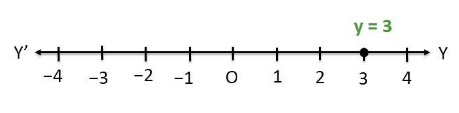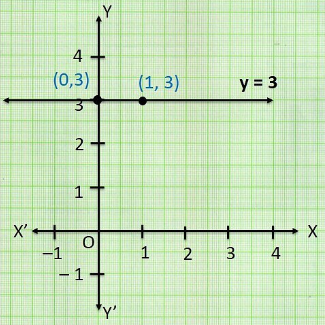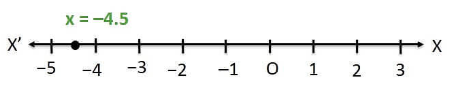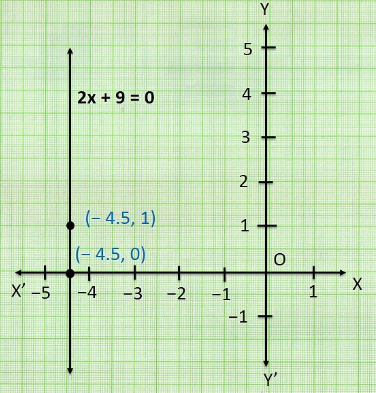# NCERT Solutions For Class 9 Math Chapter – 4 Exercise – 4.4

NCERT Solutions For Class 9 Math Chapter – 4 Exercise – 4.4

Q1. Give the geometric representation of y = 3 as an  equation

• In one variable
• In two variables

Solution:-• In one variables , it is represented as• In two variables , it is represented as a line parallel to X – axis 0x + y = 3

Q2.  Give the geometric representation of 2x + 9 = 0 as an equation

• In one variable
• In two variables

Solution:-

• In one variable , it is represented

X = -9/2• In two variables it is represented as a line parallel to Y – axis

2x + 0y + 9 = 0